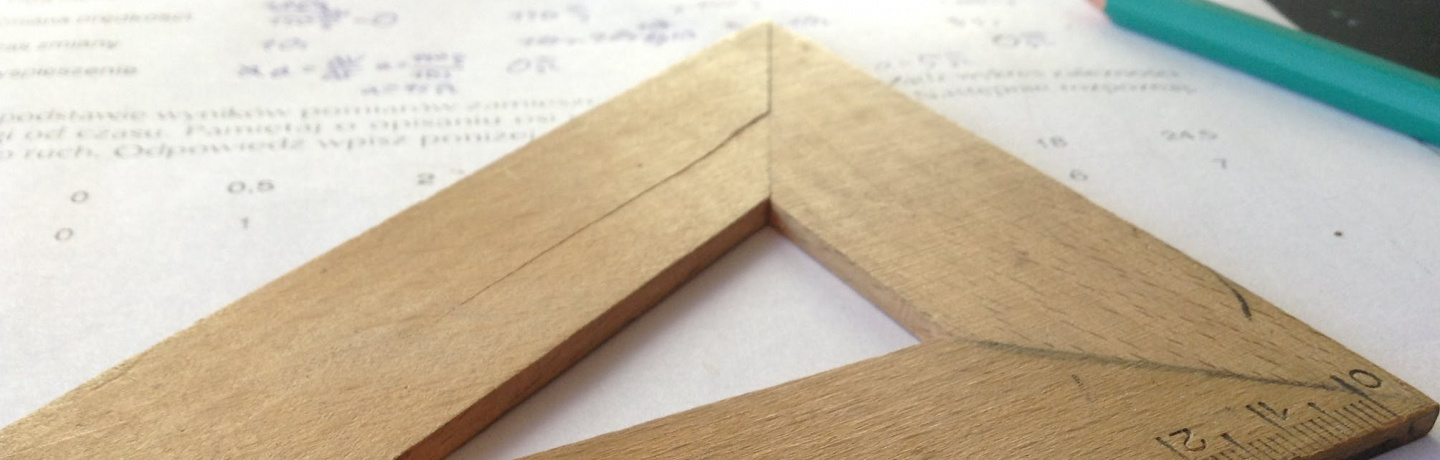# Geometric description of the Hochschild cohomology of group algebras

### online | 2020-10-01 | 14:00

Aleksandr S. Mishchenko

Universidade Estatal de Moscovo, Departamento de Geometria e Topologia, Moscovo, Rússia

There are two approaches to the study of the cohomology of group algebras $\mathbb{R}[G]$: the Eilenberg-MacLane cohomology and the Hochschild cohomology. In the case of Eilenberg-MacLane cohomology one has the classical cohomology of the classifying space $BG$. Hochschild cohomology represents a more general construction, in which so-called two-sided bimodules are considered.
The Hochschild cohomology and the usual Eilenberg-MacLane cohomology are coordinated by moving from bimodules to left modules.
For the Eilenberg-MacLane cohomology, in the case of a nontrivial action of the group $G$ in the module $M_l$, no reasonable geometric interpretation has been known so far. An effective geometric description of the Hochschild cohomology is devoted to the main result of this paper. The key point for the new geometric description of the Hochschild cohomology is the new groupoid $Gr$ associated with the adjoint action of the group $G$.
The cohomology of the classifying space $BGr$ of this groupoid with an appropriate condition for the finiteness of the support of cochains is isomorphic to the Hochschild cohomology of the algebra $\mathbb{R}[G]$. Hochschild homology is described in the form of homology groups of the space $BGr$, but without any conditions of finiteness on chains.
There is a connection between the homology of the space BGr and the cohomology of BGr in the form of an isomorphism $H^*_f(BGr,R)\approx {\bf Hom}_f(H_*(BGr), R)$ where ${\bf Hom}_f$ is a set of linear homomorphisms with a condition of finiteness.